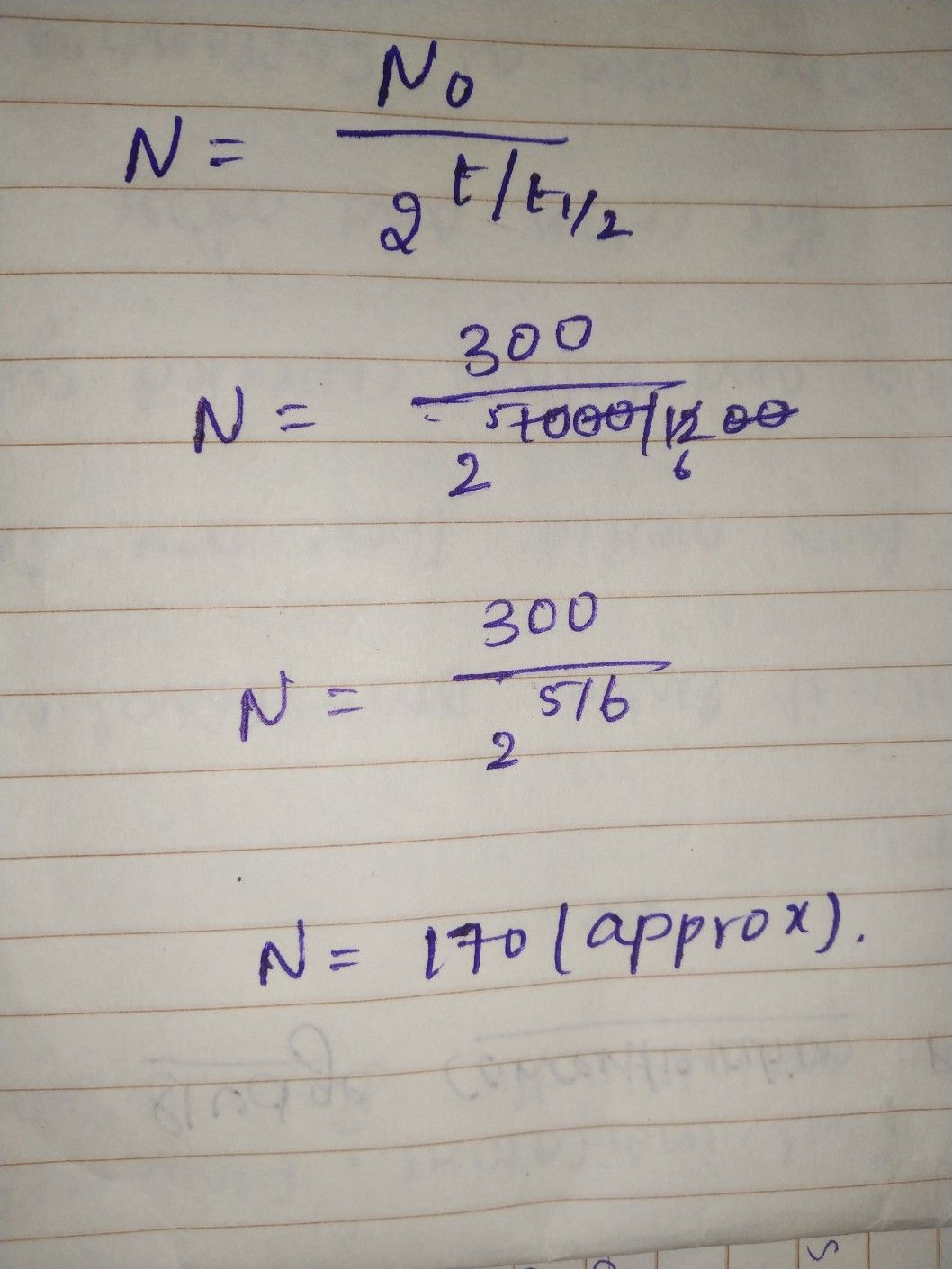Symbol
Problem$2$ The $half-life$ of a radioactive substance is $1200$ years. If the initial amount of the substance is $300$ grams, give an exponential model for the amount remaining after t years. What amount of substance remains after $1000$ years $ANsWER$
10th-13th grade
Algebra
Search count: 113
SolutionQanda teacher - RajiStudent
Can you explain please. I'm kinda confused.Qanda teacher - Raji
In the question half life of a radio active substance is given..the model given is exponential model so i used this formulaStudent
below the NO what is that? is that +/+1/2? The 2 also why is it there? Where did you get the 1200?Qanda teacher - Raji
no that was t/t 1/2Student
thank you